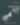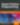# Quick Tip: Master Closures by Reimplementing Them from Scratch

JavaScript

Share:

#### Free JavaScript Book!Write powerful, clean and maintainable JavaScript.

RRP \$11.95

This article was peer reviewed by Tim Severien and Michaela Lehr. Thanks to all of SitePoint’s peer reviewers for making SitePoint content the best it can be!

To say there are a lot of articles about closures would be an understatement. Most will explain the definition of a closure, which usually boils down to a simple sentence: A closure is a function that remembers the environment in which it was created. But how does it remember? And why can a closure use local variables long after those variables have gone out of scope? To lift the veil of magic surrounding closures, I’m going to pretend that JavaScript doesn’t have closures and can’t nest functions, and then we’re going to re-implement closures from scratch. In doing so, we’ll discover what closures really are and how they work under the hood.

For this exercise, I’ll also need to pretend that JavaScript has one feature it doesn’t really have. I’ll need to pretend that an ordinary object can be called as if it were a function. You may have already seen this feature in other languages. Python lets you define a `__call__` method, and PHP has a special `__invoke` method, and it’s these methods that are executed when an object is called as if it were a function. If we pretend that JavaScript has this feature too, here’s how that might look:

``````// An otherwise ordinary object with a "__call__" method
let o = {
n: 42,
__call__() {
return this.n;
}
};

// Call object as if it were a function
o(); // 42
``````

Here we have an ordinary object that we’re pretending we can call as if it were a function, and when we do, the special `__call__` method is executed, same as if we had written `o.__call__()`.

With that, let’s now look at a simple closure example.

``````function f() {
// This variable is local to "f"
// Normally it would be destroyed when we leave "f"'s scope
let n = 42;

// An inner function that references "n"
function g() {
return n;
}

return g;
}

// Get the "g" function created by "f"
let g = f();

// The variable "n" should be destroyed by now, right?
// After all, "f" is done executing and we've left its scope
// So how can "g" still reference a freed variable?
g(); // 42
``````

Here we have an outer function `f` with a local variable, and an inner function `g` that references `f`‘s local variable. Then we return the inner function `g` and execute it from outside `f`‘s scope. But if `f` is done executing, then how can `g` still use variables that have gone out of scope?

Here’s the magic trick: A closure isn’t merely a function. It’s an object, with a constructor and private data, that we can call as if it were a function. If JavaScript didn’t have closures and we had to implement them ourselves, here’s how that would look.

``````class G {
// An instance of "G" will be constructed with a value "n",
// and it stores that value in its private data
constructor(n) {
this._n = n;
}

// When we call an instance of "G", it returns the value from its private data
__call__() {
return this._n;
}
}

function f() {
let n = 42;

// This is the closure
// Our inner function isn't really a function
// It's a callable object, and we pass "n" to its constructor
let g = new G(n);

return g;
}

// Get the "g" callable object created by "f"
let g = f();

// It's okay if the original variable "n" from "f"'s scope is destroyed now
// The callable object "g" is actually referencing its own private data
g(); // 42
``````

Here we replaced the inner function `g` with an instance of the class `G`, and we captured `f`‘s local variable by passing it to `G`‘s constructor, which then stores that value in the new instance’s private data. And that, ladies and gentlemen, is a closure. It really is that simple. A closure is a callable object that privately stores values passed through the constructor from the environment in which it was instantiated.

## Taking It Further

The astute reader will notice there’s some behavior we haven’t yet accounted for. Let’s look at another closure example.

``````function f() {
let n = 42;

// An inner function that references "n"
function get() {
return n;
}

// Another inner function that also references "n"
function next() {
n++;
}

return {get, next};
}

let o = f();

o.get(); // 42
o.next();
o.get(); // 43
``````

In this example, we have two closures that both reference the same variable `n`. One function’s manipulation of that variable affects the other function’s value. But if JavaScript didn’t have closures and we had to implement them ourselves, then we wouldn’t get that same behavior.

``````class Get {
constructor(n) {
this._n = n;
}

__call__() {
return this._n;
}
}

class Next {
constructor(n) {
this._n = n;
}

__call__() {
this._n++;
}
}

function f() {
let n = 42;

// These are the closures
// They're callable objects that privately store the values
// passed through their constructors
let get = new Get(n);
let next = new Next(n);

return {get, next};
}

let o = f();

o.get(); // 42
o.next();
o.get(); // 42
``````

Like before, we replaced the inner functions `get` and `next` with instances of the classes `Get` and `Next`, and they capture `f`‘s local variable by passing it to the constructors and storing that value in each instance’s private data. But notice that one callable object’s manipulation of `n` didn’t affect the other callable object’s value. This happened because they didn’t capture a reference to `n`; they captured a copy of the value of `n`.

To explain why JavaScript’s closures will reference the same `n`, we need to explain variables themselves. Under the hood, JavaScript’s local variables aren’t really local in the traditional sense. Instead, they’re properties of a dynamically allocated and reference counted object, called a “LexicalEnvironment” object, and JavaScript’s closures capture a reference to that whole environment rather than to any one particular variable.

Let’s change our callable object implementation to capture a lexical environment rather than `n` specifically.

``````class Get {
constructor(lexicalEnvironment) {
this._lexicalEnvironment = lexicalEnvironment;
}

__call__() {
return this._lexicalEnvironment.n;
}
}

class Next {
constructor(lexicalEnvironment) {
this._lexicalEnvironment = lexicalEnvironment;
}

__call__() {
this._lexicalEnvironment.n++;
}
}

function f() {
let lexicalEnvironment = {
n: 42
};

// These callable objects capture a reference to the lexical environment,
// so they will share a reference to the same "n"
let get = new Get(lexicalEnvironment);
let next = new Next(lexicalEnvironment);

return {get, next};
}

let o = f();

// Now our callable objects exhibit the same behavior as JavaScript's functions
o.get(); // 42
o.next();
o.get(); // 43
``````

Here we replaced the local variable `n` with a `lexicalEnvironment` object that has a property `n`. And the closures—the callable instances of classes `Get` and `Next`—capture a reference to the lexical environment object rather than the value of `n`. And because they now share a reference to the same `n`, one callable object’s manipulation of `n` affects the other callable object’s value.

## Conclusion

Closures are objects that we can call as if they were functions. Every function in JavaScript is in fact a callable object, also called a “function object” or “functor”, that is instantiated with and privately stores a lexical environment object, even if it’s the outermost global lexical environment. In JavaScript, a function doesn’t create a closure; the function is the closure.

Has this post helped you to understand closures? I’d be glad to hear your thoughts or questions in the comments below.### Jeff Mott

Jeff has been doing frontend and backend Web development since '98 when Perl and floppy disks were a thing. Later he worked in PHP and Symfony with an agency in San Francisco. In 2009, he joined Intel and often works with NodeWebkit, Angular, C++, Python, and much more.

#### New books out now!Learn how Git works, and how to use it to streamline your workflow!Google, Netflix and ILM are Python users. Maybe you should too?